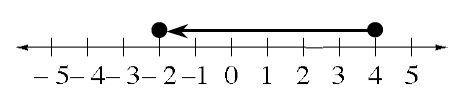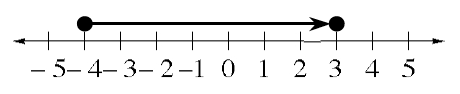### Home > CCAA > Chapter 2 Unit 2 > Lesson CC2: 2.2.2 > Problem2-51

2-51.

Write an expression to represent each of the following sketches. Then give the value of each sketch.

1. $\array{+}\array{+\\+\\- }\array{+\\-\\+}\array{-\\ \\ \\}$

Look for pairs of positive and negative integers that will add to zero.

This will be easier if you line up the symbols, as below.

#### $\array{{\enclose{circle}{+++\\---}}++}$

Write an expression representing the integers that added to zero, plus the remaining integers.

$0+0+0+1+1$

One solution to this problem is $0+0+0+1+1=2$.
You can also write this as $5+(-3)=2$.

1.Where does the arrow begin? Where does it end? How long is the arrow?

$4-6=-2$ or $4+(-6)=-2$

1. $\array{-\\ }{++-\\+--+}$

How many $+$'s are there? How many $-$'s? How many zeros? When you eliminate the zeros, what is left over?

1.See the help for part (b).